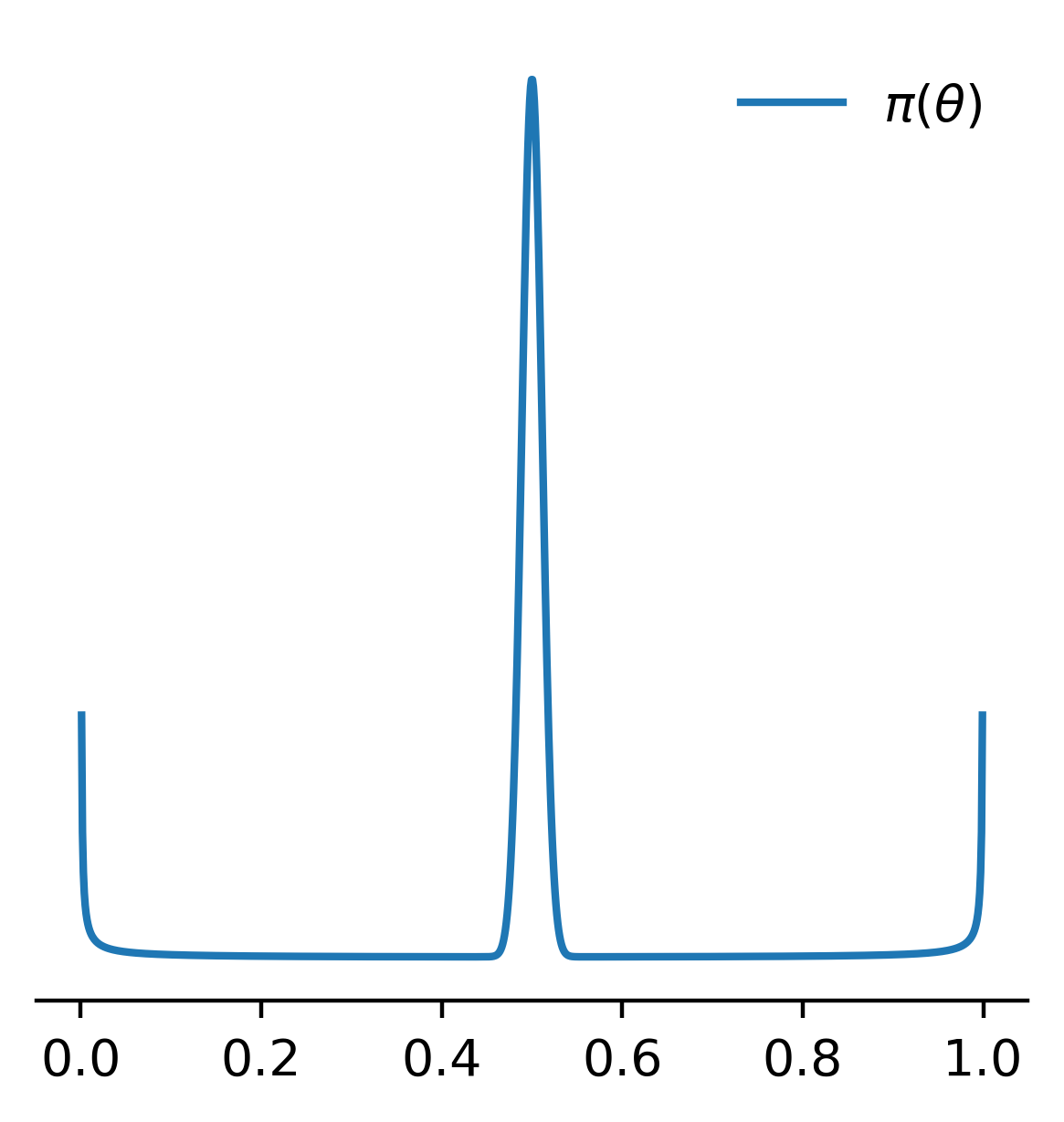# Approximate Bayesian Computation and Insurance¶

## Patrick J. Laub and Pierre-Olivier Goffard¶

### https://pat-laub.github.io/talks/abc¶## Motivation¶

Have a random number of claims $N \sim p_N( \,\cdot\, ; \boldsymbol{\theta}_{\mathrm{freq}} )$ and the claim sizes $U_1, \dots, U_N \overset{\mathrm{i.i.d.}}{\sim} f_U( \,\cdot\, ; \boldsymbol{\theta}_{\mathrm{sev}} )$.

We aggregate them somehow, like:

• aggregate claims: $X = \sum_{i=1}^N U_i$
• maximum claims: $X = \max_{i=1}^N U_i$
• stop-loss: $X = ( \sum_{i=1}^N U_i - c )_+$.

Question: Given a sample $X_1, \dots, X_n$ of the summaries, what is the $\boldsymbol{\theta} = (\boldsymbol{\theta}_{\mathrm{freq}}, \boldsymbol{\theta}_{\mathrm{sev}})$ which explains them?

Easier question: Given $(X_1, N_1), \dots, (X_n, N_n)$ summaries & counts, what is $\boldsymbol{\theta}$?

## Likelihoods¶

For simple rv's we know their likelihood (normal, exponential, gamma, etc.).

When simple rv's are combined, the resulting thing rarely has a likelihood.

$$X_1, X_2 \overset{\mathrm{i.i.d.}}{\sim} f_X(\,\cdot\,) \Rightarrow X_1 + X_2 \sim ~ \texttt{Unknown Likelihood}!$$

For a sample of $n$ i.i.d. observations the joint likelihood is

$$p_{\boldsymbol{X}}(\boldsymbol{x} \mid \boldsymbol{\theta}) = \prod_{i=1}^n p_{X_i}(x_i; \boldsymbol{\theta}) \,.$$

If $n$ increases, then $p_{\boldsymbol{X}}(\boldsymbol{x} \mid \boldsymbol{\theta}) = \prod \text{Small things} \overset{\dagger}{=} 0$, or just takes a long time to compute, then $\texttt{Intractable Likelihood}$!

## Bayesian statistics¶Prior distribution $\pi(\boldsymbol{\theta})$

Likelihood $\pi( \boldsymbol{x} \mid \boldsymbol{\theta} )$

Posterior distibution

$$\pi(\boldsymbol{\theta} \mid \boldsymbol{x} ) = \frac{ \pi(\boldsymbol{\theta}) \pi( \boldsymbol{x} \mid \boldsymbol{\theta} ) }{ \pi( \boldsymbol{x} ) }$$

For further reading, see Frequentists vs. Bayesians (xkcd).

## Markov chain Monte Carlo¶## Approximate Bayesian Computation¶

Example: Flip a coin a few times and get $(X_1, X_2, X_3) = (\text{H, T, H})$; what is $\theta = \mathbb{P}(\text{Heads})$?

## Exact matching algorithm¶

Given some observations $\boldsymbol{x}_{\text{obs}}$, repeat:

• generate a potential parameter from the prior distribution $\boldsymbol{\theta}^{\ast} \sim \pi(\boldsymbol{\theta})$;
• simulate some 'fake data' $\boldsymbol{x}^{\ast}$ from the model $\boldsymbol{\theta}^{\ast}$;
• if $\boldsymbol{x}_{\text{obs}} = \boldsymbol{x}^{\ast}$, then store $\boldsymbol{\theta}^{\ast}$.

The resulting $\boldsymbol{\theta}^{\ast}$s are an i.i.d. sample from the posterior $\pi(\theta \mid \boldsymbol{x}_{\text{obs}})$.